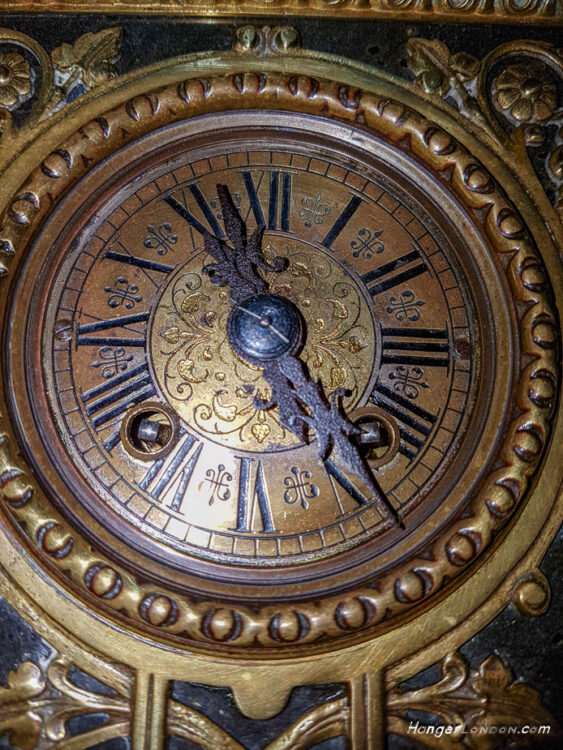# 1925 Daylight Saving Act came into force in Britain

British Summer time was the term used that meant the clocks had to be changed twice a year.

1 hour forwards “Spring Forwards in Spring”   or

backwards “Fall Backwards” when the leaves fall in Autumn

25th March 2018 and 28th October 2018.

https://youtu.be/-YJQs588OTQ

Article Rating
Subscribe
Notify of
Inline Feedbacks

## Maths Quiz: Test your basic Maths

/9
10
Created on

Mathematic Quiz - Prime and Composite Numbers

Math quiz helps us to increase our knowledge

1 / 9

is 29 PRIME number or a COMPOSITE number?

2 / 9

is 61 a Prime number or a Composite number?

3 / 9

is 850 PRIME number or a COMPOSITE number?

4 / 9

is 16 PRIME number or a COMPOSITE number?

5 / 9

is 601 PRIME number or a COMPOSITE number?

6 / 9

is 441 PRIME number or a COMPOSITE number?

7 / 9

is 987 PRIME number or a COMPOSITE number?

8 / 9

is 908 PRIME number or a COMPOSITE number?

9 / 9

is 529 PRIME number or a COMPOSITE number?